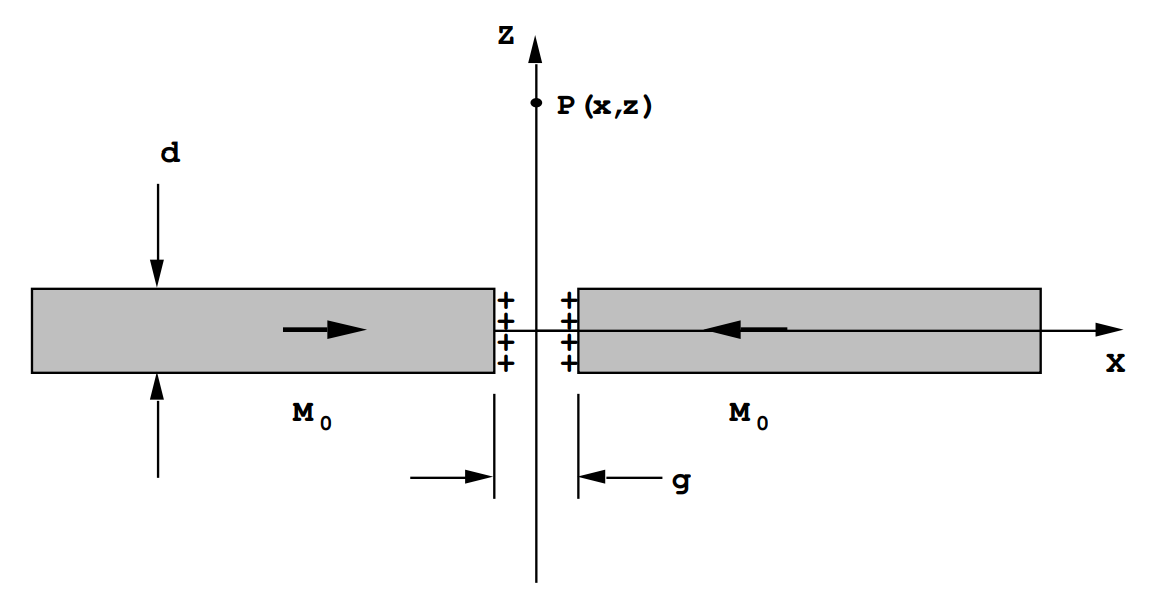$$\require{cancel}$$

# 13.6: Chapter 6

Problem (6.1)

An electromagnet is constructed of a soft iron yoke, see the diagram. The yoke radius is R= 2.54 cm, and the gap is d= 1.25 cm. The distance from pole face to pole face along the dotted line is L= 55 cm. The number of turns on the coil is 1000 windings. Estimate the current required to generate a field of 1.0 Teslas at the center of the magnet gap. A field of 1.0 T in the iron yoke corresponds to a field H of 130 Amps/m.$$\oint_{C} \mathbf{H} \cdot \text{d} \mathbf{L}=\text{NI}$$

Therefore, 130L + d/µ0 = NI.

or

$$\begin{array}{l} \text { (13) }(0.55)+(0.0125) /\left(4 \pi \times 10^{-7}\right)=10^{3} \text{I} \end{array}$$,

and

I= 10.0 Amps.

Problem (6.2)

Modern permanent magnet materials such as FeNdB can be used to generate substantial magnetic fields. Consider the configuration shown in the diagram where the cross-hatched regions represent FeNdB permanent magnets.The saturation magnetization density in each magnet is M0= 0.8x106 Amps/m., ie. B= µ0M0 = 1.01 Teslas. Let R=L= 1.0 cm., and let d= 1/2 cm.

Calculate the field B at the midpoint of the gap. The approximate effect of the iron yoke is to make each permanent magnet appear to be infinitely long due to the magnetization induced in the soft iron. In soft iron having a very large permeability the magnetization must be continuous at the iron-magnet interface because a discontinuity in M would produce an H- field which would produce a large M in the iron and as a result B would not be continuous.

The field generated at the gap center can be approximated using a superposition argument. If there were no gap the field would be that due to an infinitely long solenoid having NI= M0, ie. B= µ0M0.

The field in the gap,BG, plus the field at the center due to a magnetized section 2d long must equal µ0M0. A section 2d long possessing a magnetization density M0 produces a field at its center given by the short solenoid formula $$B_{s}=\frac{\mu_{0} M_{0} d}{\sqrt{d^{2}+R^{2}}}$$. Therefore

$\text{B}_{\text{G}}+\text{B}_{\text{S}}=\mu_{0} \text{M}_{0},\nonumber$

and

$\text{B}_{\text{G}}=\mu_{0} \text{M}_{0}\left(1-\frac{\text{d}}{\sqrt{\text{d}^{2}+\text{R}^{2}}}\right).\nonumber$

In this case d=1/2 cm and R=1 cm, so BG= 0.558 Teslas.

R. Oldenbourg and W.C. Phillips,

(Rev.Sci.Instrum.57,2362(1986) and 57,3139(1986)), used 2d=1.9 cm and soft iron pole tips tapered to 0.35 cm in diameter to produce a field of 2 Teslas in a 0.2 cm gap.

Problem (6.3)

This problem has to do with the fields produced in the region between two magnetized bits on a hard disc, see the figure. The gap is g= 10-8 meters, the thickness is d= 10-8 meters, and the width of each magnetized region is w= 0.4x10-6 meters. the magnetization is M0= 6.4x105 Amps/m.The end of each magnetized region bears a surface charge of M0 per square meter due to the discontinuity in the magnetization density.

Calculate the field at P(x,o,z) on the centerline of the gap between the two magnetized regions due to the magnetic surface charges.

On the centerline H has only a z-component by symmetry.

Let r= (g/2)ux + yuy + zuz,

and R= Zuz.

The vector from the element of magnetic charge dq=dydzM0 to the point of observation is $$\rho=\mathbf{R}-\mathbf{r}=-(g / 2) \mathbf{u}_{\mathbf{X}}-y \mathbf{u}_{\mathbf{Y}}+(Z-z) \mathbf{u}_{\mathbf{z}}$$, and $$|\rho|=\sqrt{(g / 2)^{2}+y^{2}+(Z-z)^{2}}$$.

For one of the end faces

$\text{H}_{\text{z}}=\frac{\text{M}_{0}}{4 \pi} \int_{-\text{d} / 2}^{\text{d} / 2} \text{d} \text{z}(\text{z}-\text{z}) \quad \int_{-\text{W} / 2}^{\text{W} / 2} \text{d} \text{y} \frac{1}{\left(\text{y}^{2}+(\text{g} / 2)^{2}+(\text{Z}-\text{z})^{2}\right) 3 / 2}\nonumber.$

Now

$\int_{-\text{w} / 2}^{\text{w} / 2} \frac{\text{d} \text{y}}{\left(\text{y}^{2}+\text{a}^{2}\right)^{3 / 2}}=\frac{\text{w}}{\text{a}^{2} \sqrt{(\text{w} / 2)^{2}+\text{a}^{2}}}\nonumber,$

therefore

$\text{H}_{\text{z}}=\frac{\text{M}_{0} \text{w}}{4 \pi} \int_{-\text{d} / 2}^{\text{d} / 2} \text{d} \text{z} \frac{(\text{Z}-\text{z})}{\left((\text{g} / 2)^{2}+(\text{Z}-\text{z})^{2}\right) \sqrt{(\text{Z}-\text{z})^{2}+(\text{g} / 2)^{2}+(\text{w} / 2)^{2}}}\nonumber$

Let v= (Z-z)2+(g/2)2, then the integral becomes

$\mathrm{H}_{\mathrm{z}}=\frac{\mathrm{M}_{0} \mathrm{w}}{8 \pi} \int_{(\mathrm{Z}-\mathrm{d} / 2)^{2}+(\mathrm{g} / 2)^{2}}^{(\mathrm{Z}+\mathrm{d} / 2)^{2}+(\mathrm{g} / 2)^{2}} \mathrm{d} \mathrm{v} \quad \frac{1}{\mathrm{v} \sqrt{\mathrm{v}+(\mathrm{w} / 2)^{2}}}.\nonumber$

This is a standard integral:

$\text{H}_{\text{z}}=\frac{\text{M}_{0}}{2 \pi}\left\{\tanh ^{-1}\left[\frac{2}{\text{w}} \sqrt{(\text{w} / 2)^{2}+(\text{g} / 2)^{2}+(\text{Z}-\text{d} / 2)^{2}}\right]\right.\left.\left.-\tanh ^{-1}\left[\frac{2}{w} \sqrt{(w / 2)^{2}+(g / 2)^{2}+(z+d / 2)^{2}}\right)\right]\right\}\nonumber.$

(This is for one face of the gap magnetic charge distribution- it must be multiplied by 2 to obtain the total field).

For (w/2)=20x10-8, (g/2)=(1/2)x10-8, and (d/2)= (g/2), and if Z= 1.0x10-8 meters Bz= µ0Hz= 0.206 Teslas. For the above parameters Bz is a maximum for Z=0.71x10-8 meters. The maximum value of Bz= 0.224 T. At Z=2x10-8m the field has dropped to Bz= 0.121 T.